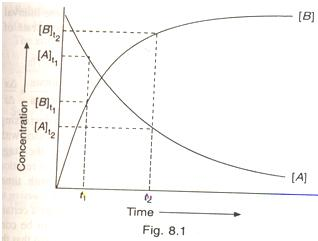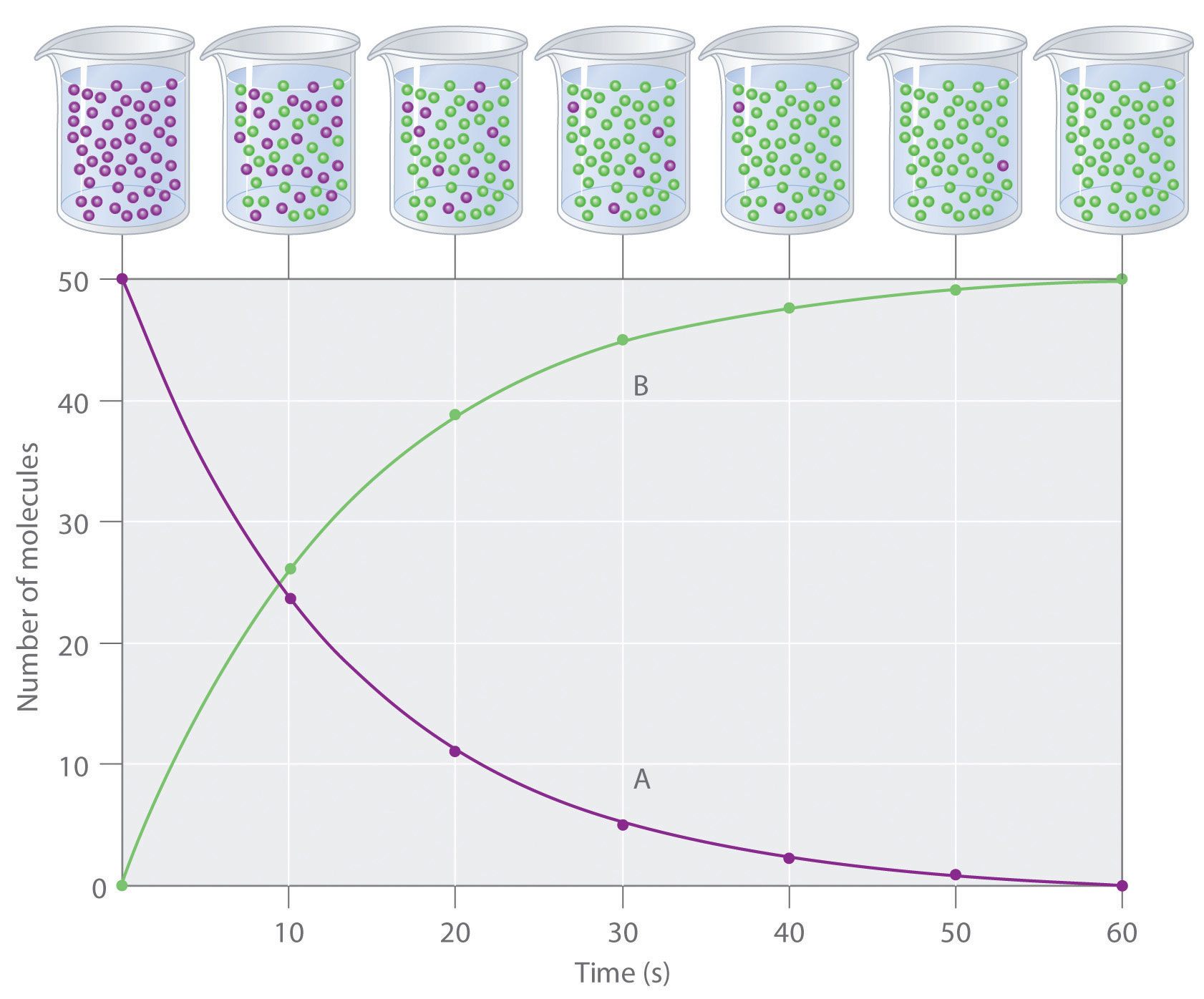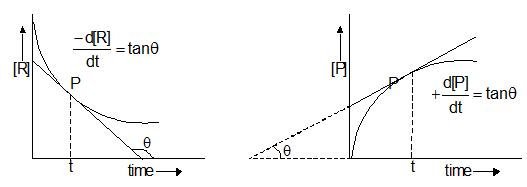×#### Thank you for registering.

One of our academic counsellors will contact you within 1 working day.

Click to Chat

1800-1023-196

+91-120-4616500

CART 0

• 0

MY CART (5)

Use Coupon: CART20 and get 20% off on all online Study Material

ITEM
DETAILS
MRP
DISCOUNT
FINAL PRICE
Total Price: Rs.

There are no items in this cart.
Continue ShoppingRate of Reaction

Table of Content

Average Rate of Reaction

Expression of Rate

Instantaneous Rate

Reaction Life Time

Rate Constant

Unit of Rate Constant

Difference between Rate of Reaction and Rate Constant

Related Resources

​The change in the number of moles / litre of any reactant or product per unit time is known as rate of reaction.

The rate measured over a long time interval is called average rate and the rate measured for an infinitesimally small time interval is called instantaneous rate.

In a chemical change, reactants and products are involved. As the chemical reaction proceeds, the concentration of the reactants decreases, i.e., products are produced.

The rate of reaction (average rate) is defined as the change of concentration of any one of its reactants (or products) per unit time.Average Rate of Reaction

Consider a common hypothetical reaction,

A → B

The concentration of reactants or products are expressed as number of moles per litre. The symbol for the molar concentration consists of the formula of the substance enclosed in bracket. The symbol [A] stands for the molar concentration of A. the symbol ?[A], therefore, stands for the change in molar concentration of A.

The rate if decrease or increase in the concentration of reactants or products may also be expressed in terms of change in their concentration during the time interval, ?t, as

Average rate of reaction =

Average rate of reaction =

The average rate of reaction may be expressed either in terms of decrease in concentration of A (reactants) or in terms of increase in concentration of B (product).

Average rate of reaction= (Decrease in concentration of A)/(Time taken)

Average rate of reaction= (Increase in concentration of B)/(Time taken)

So in general,

or

Where DC is change in the concentration of reactant or product during time interval Dt.

=  rate at which concentration of product increases

=rate at which concentration of reactant decreases

A negative sign placed before a reaction rate symbol signifies a decrease in concentration of the reactant with increase of time and a positive sign before the rate symbol signifies that the concentration of the product increases with increase in time. The concentration change may be positive or negative but the rate of reaction is always positive. The minus sign is always written when required but the plus sign is usually not mentioned.

Rate of reaction is not uniform. It goes on decreasing from moment to moment due to decrease in the concentration(s) of reactant(s) with the progress of reaction i.e. with time as shown below by rate vs time curve. Thus, the rate defined above is actually the average rate of reaction during the time interval considered.

Figure 8.1 show the change of concentrations of reactant (A) and product (B) as the chemical reaction A → B progresses with time. Let  and  be the concentration of A at time t1 and t2, respectively.

?[A] = [A]t2-[A]t1

and    ?t = t2 - t1Over the same time interval t1 and t2, the concentration of B changes from [B]t1 to [B]t2.

Since one mole of A produces one mole of B, the rate of decrease in concentration of A should be equal to the rate of increase in concentration of B. Thus,

Rav = R'av

Reaction rate has the units of concentration or molarity divided by time.

Units of Rate of Reaction:

mole/litre sec (mol L-1 s-1)

mole/litre min (mol L-1 min-1)

mole/litre hour (mol L-1 h-1)

mole/litre day (mol L-1 d-1)

mole/litre year (mol-1y-1 )

Expression of Rate

For the reaction:

2N2O5  →  4NO2 + O2

the rate can be expressed as one of the following ways

These individual rates may be made equal to one another by dividing them by the respective stoichiometric coefficients in the chemical equation for the reaction. Thus,

Rate of reaction

Instantaneous Rate

In chemical kinetic, the rate at any particular instant, i.e., instantaneous rate rather than the average rate over a time interval has much more practical application and importance. It is defined as the rate of change of concentration of any one of the reactants or products over a very small interval of time.

If we take infinitesimal small interval of time dt, it may be assumed that the rate is uniform through this interval; then if dx is amount of substance A transformed to B bring this interval, the rate of reaction at that instant is given as .

In differential calculus, when Δt becomes very small and approaches zero, the ratio

may be replaced by the derivative,  . that is

On the other hand, if the rate of reaction is expressed in terms of the concentration of any of the products which goes on increasing, then rate of reaction at particular instant = dx/dt.

In general, for a reaction,

n1A + n2B → m1C + m2D

The instantaneous rate may be expressed as

rinst = 1/n1 d{A)/dt = -1/n2 = d(B)/dt = 1/m1 d(C)/dt=1/m2 d(D)/dt

Experimental determination of instantaneous rate of reaction

The rate of reaction at any time t may be determined by plotting concentration (of reactant or product) vs. time and drawing a tangent at the point P of the curve corresponding to time t at which rate is to be determined. The slope of the tangent gives rate of reaction at the required time.([R] = concentration of reactant and [P] = concentration of product)

Rate at the time t = slope of tangent = tan

Reaction Life Time

It is defined as the time taken by a reaction to proceed to 98% of completion.

The shorter the life time, the faster the reaction.

Reaction life times are used to compare the various reaction.

Reactions are also compared with half life periods.

The half life period is defined as the time during which the concentration of a reactant is reduced to one half of its initial value or the time in which half of the reaction is completed. It is generally denoted by t1/2­. The shorter the half life period, the faster is the reaction.

Rate Constant

Refer to the following video for rate law and rate constant

Consider a simple reaction

A → B.

If CA is the molar concentration or active mass of A at a particular instant, then

where k is a proportionality constant, called velocity constant or rate constant or specific reaction rate.

At a fixed temperature, if CA = 1, then

….(i)

Let us consider a general reaction.

aA + bB → product

When

[A] = [B] = 1 mol/litre, then

Rate = K

Rate of a reaction at unit concentration of reactants is called rate constant.

The value of rate constant depends on:

Nature of reactant

Temperature

Catalyst

Unit of Rate Constant

Rate constant has different units for reactions of different order. General rule for rate of reaction may be given as:

where n = order of reaction

Difference between Rate of Reaction and Rate Constant

S.No.

Rate of reaction

Reaction rate constant

1
It is speed with which reactants are converted into products.
It is proportionally constant.

2
It is measured as the rate of decrease of concentration of reactants or the rate of increase of concentration of products with time.
It is equal to the rate of reaction when the concentration of each of the reactants in unity.

3
It depends upon the initial concentration of reactants
It is independent of the initial concentration of the reactants. It has a constant value at fixed temperature.

Solved Example

Example 1  :

The following reaction was carried out in water : Cl2 + 2I- → I2 + 2Cl-

The initial concentration of I- was 0.25 mol L-1 and the concentration after 10 minutes 0.23 mol L-1. Calculate the rate of disappearance of I- and appearance of I2.

Solution  :

?[I-]  =  [I-]final - [I-]initial = 0.23 - 0.25 = -0.02 mol L-1

?t = 10 - 0 = 10 min

-?[I- ]/?t = -((-0.02))/10 = 0.002  mol L-1 min-1

Rate of appearance of I2  = 1/2 ?[I- ]/?t = 0.002/2

= 0.001 mol L-1 min-1

= 1 × 10-3 mol L-1 min-1

Example 2  :

The reaction between Cr2O72- and HNO2 in an acidic medium is Cr2O72- + 15H+ + 3HNO2 → 2Cr3+ + 3NO3- + 4H2O

The rate of disappearance of  is found to be 2.4 × 10-4 mol L-1 s-1 during a measured time interval. Find the rate of disappearance of HNO2 and the rate of appearance of Cr3+ during thus time interval.

Solution  :

The equality in this case is

-?[Cr2O72-]/?t = 1/3 ?[HNO2 ]/?t = 1/2(?[Cr3+])/?t

It is given that

-?[Cr2O72- ]/?t = 2.4×10-4   mol L-1 s-1

So -?[HNO2]/?t = 3×2.4×10-4 = 7.2×10-4   mol L-1 s-1

and ?[Cr3+]/?t 2×2.4×10-4 = 4.8×10-4   mol L-1 s-1Question 1: As the chemical reaction proceeds, the concentration of

a. reactants increases

b. prducts increases

c. reactant remains constant

d. products decreases

Question 2: Rate of reaction is rate of change of concentration with

a. pressure

b. temperature

c. volume

d. time

Question 3: Which of the following expressions is correct for rate of reaction  HNO2 → 2Cr3+ + 3NO3- + 4H2O

a. d[HNO2]/dt

b. d[2Cr3+]/dt

c. -d[4H2O]/dt

d. -d[3NO3-]/dt

Question 4: It is defined as the time taken by a reaction to proceed to 98% of completion is known as

a. half life of reaction

b. rate of reaction

c. reaction life time

d. reaction coefficientQ.1
Q.2
Q.3
Q.4

b
d
b
c

Related Resources

Look here for  Useful Books of Chemistry

You can also refer to Introduction to chemical kinetics

To read more, Buy study materials of Chemical Kinetics comprising study notes, revision notes, video lectures, previous year solved questions etc. Also browse for more study materials on Chemistry here.### Course Features

• 731 Video Lectures
• Revision Notes
• Previous Year Papers
• Mind Map
• Study Planner
• NCERT Solutions
• Discussion Forum
• Test paper with Video Solution